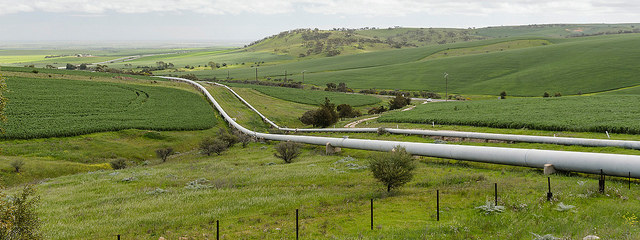# Mongo DB Aggregation vs R dplyrThere are powerful options to do the processing you want:

1. Aggegation operations process data records results. Aggregation operations group values from multiple documents together, and can perform a variety of operations on the grouped data to return a single result.

2. dplyr framework in R

dplyr is a grammar of data manipulation, providing a consistent set of verbs to solve most common data manipluation tasks. The framework is part of the tidyverse ecosystem in R.

In this post I want to shortly compare the code needed for aggregated values site-by-site on a practial example on which I worked recently on: Airbnb Local Market Analysis. In this projects live calendar data for all Airbnb listings for a region are scraped from their website and persisted into a MongoDB. For every day in the calendar there is one document per listing in the database, that looks like this:

{
"_id" : ObjectId("5b113b287c9f8e50597db3a6"),
"available" : false,
"loc" : {
"type" : "Point",
"coordinates" : [
114.139377439,
22.2862727991
]
},
"price" : {
"local_price" : NumberInt(57),
"native_price" : 510.0,
"date" : "2018-04-29",
"native_currency" : "HKD",
"local_currency" : "EUR",
"type" : "default",
"local_price_formatted" : "€57"
},
"max_nights" : NumberInt(7),
"date" : ISODate("2018-04-29T00:00:00.000+0000"),
"min_nights" : NumberInt(2),
"id" : NumberInt(10002239)
}


From the calendar data different metrics needed to be calculated: E.g. Occupancy which is the proportion a listing is booked out in a time period, or the average price a night is sold.

This is a typical scenario for calculating summary statistics from raw data.

## Mongo DB aggregation framework:

Aggregation pipelines consist of different stages. Let’s build the pipeline stage by stage:

• First we want to fetch all listings based on their geolocation. We want all the dates for all listing in an area 100,000 meters around a geo location:
{
"$geoNear": { "near": { "type": "Point", "coordinates": [ 10.9867379057, 49.4650778012 ] }, "spherical": 1, "maxDistance": 100000, "distanceField": "dist", "limit": 100000 } }  • Next two helper fields are added. One is the ‘paid_price’, which is price that was paid for a booking and 0 in case of a place not booked. The field ‘unavailable’ is needed as a helper field for calculation the number of unavailable days by replacing the boolean values with binary values. { '$addFields': {
'paid_price': {
'$cond': { if: {$eq: [
"$available", false ] }, then: '$price.local_adjusted_price',
else: 0
}
},
'unavailable': {
'$cond': { if: {$eq: [
"$available", false ] }, then: 1, else: 0 } } } } }  • Next we group on the listing id and calculate helper variables for preperation of the final results. We calculate total revenue by adding up alle the paid prices, the number of all days for the group and the number of unavailable days: { '$group': {
'_id': '$id', 'total_revenue': { '$sum': '$paid_price' }, 'num_unavailable': { '$sum': '$unavailable' }, 'num_total': { '$sum': 1
}
}
}

• Finally we calculate the metrics occupancy and average daily rate
{
'$project': { 'occupancy': {$divide: [
"$num_unavailable", '$num_total'
]
},
'average_daily_rate': {
$divide: [ "$total_revenue",
'$num_unavailable' ] } } }  The final result: [ { "$geoNear": {
"near": {
"type": "Point",
"coordinates": [
10.9867379057,
49.4650778012
]
},
"spherical": 1,
"maxDistance": 100000,
"distanceField": "dist",
"limit": 100000
}
},
{
'$addFields': { 'paid_price': { '$cond': {
if: {
$eq: [ "$available",
false
]
},
then: '$price.local_adjusted_price', else: 0 } }, 'unavailable': { '$cond': {
if: {
$eq: [ "$available",
false
]
},
then: 1,
else: 0
}
}
}
},
{
'$group': { '_id': '$id',
'total_revenue': {
'$sum': '$paid_price'
},
'num_unavailable': {
'$sum': '$unavailable'
},
'num_total': {
'$sum': 1 } } }, { '$project': {
'occupancy': {
$divide: [ "$num_unavailable",
'$num_total' ] }, 'average_daily_rate': {$divide: [
"$total_revenue", '$num_unavailable'
]
}
}
}
]


## dpylr Framework

With R we need to fetch the dates for the listings we are interested in. We could do this by simple fetch everything from the MongoDB and do everything with dplyr.

In this example we fetch the dates for all the listing near a location. We actually use the first stage from our pipeline on our MongoDB pipeline.

pipeline <- '[
{
"$geoNear": { "near": { "type": "Point", "coordinates": [ 10.9867379057, 49.4650778012 ] }, "spherical": 1, "maxDistance": 100000, "distanceField": "dist", "limit": 100000 } }]' dates.collection <- mongo("dates", "airbnb", url = "mongodb://localhost:27017") dates.df <- flatten(dates.collection$aggregate(pipeline))


Next we do the aggregation on the dates dataframe with dplyr:

dates.df %>%
group_by(id) %>%
summarize(
occupancy = sum(available == FALSE) / n(),
/ sum(available == FALSE)
)


Wow, this very simple and readable. Indeed it’s simplier than in a Aggregation pipeline in MongoDB.

But we cheated a little bit, as the initial loading is done with an easy 1-stage-aggregation-pipeline in Mongo.

library(tidyverse)
library(jsonlite)
library(mongolite)
library(glue)

pipeline <- '[
{
"$geoNear": { "near": { "type": "Point", "coordinates": [ 10.9867379057, 49.4650778012 ] }, "spherical": 1, "maxDistance": 100000, "distanceField": "dist", "limit": 100000 } }]' dates.collection <- mongo("dates", "airbnb", url = "mongodb://localhost:27017") dates.df <- flatten(dates.collection$aggregate(pipeline))

dates.df %>%
group_by(id) %>%
summarize(
occupancy = sum(available == FALSE) / n(),
/ sum(available == FALSE)
)



## Conclusion

Both options for aggregation of new data are pretty straightforward and actually similiar.

I would prefer the option with dplyr, because it’s simple and I like to have all complicated calculations and aggregations in my data analysis code. I don’t want to switch from MongoDB and R and back all the time.

It’s defintely worth your while to learn the aggregation framework from MongoDB as you can use it together (like we did with the geonear search) with dplyr.